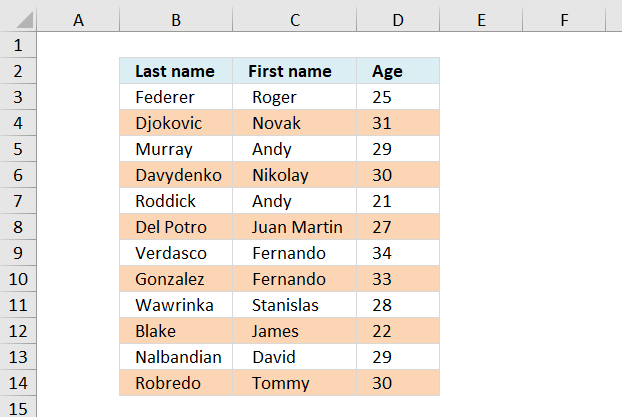Author: Oscar Cronquist Article last updated on October 13, 2018Here is how to highlight every other row using conditional formatting.

Conditional formatting formula:

=ISEVEN(ROW())*OR(\$B3:\$D3<>"")

Alternative CF formula:

=EVEN(ROW())=ROW()

This formula colors every second row if any cell in the row is populated. If you know you will add more records later to the list, select and conditional format a range larger than the list. Empty rows won´t be formatted.

How to apply the conditional formatting formula in excel 2007:

1. Select the range, example cell range A:C
2. Click "Home" tab on the ribbon
3. Click "Conditional formatting"
4. Click "New rule..."
5. Click "Use a formula to determine which cells to format"
6. Click "Format values where this formula is true" window.
7. Type =ISEVEN(ROW())*OR(\$B3:\$D3<>"")
8. Click Format button
9. Click "Fill" tab
10. Select a color
11. Click OK!
12. Click OK!

### Explaining the contional formatting formula in cell A2

=ISEVEN(ROW())*OR(\$A2:\$C2<>"")

Step 1 - Check if row number is even

=ISEVEN(ROW())*OR(\$A2:\$C2<>"")

ISEVEN(ROW())

becomes

ISEVEN(2)

returns TRUE in cell A2.

Step 2 - Check if  any of the cells in row are not empty

=ISEVEN(ROW())*OR(\$A2:\$C2<>"")

OR(\$A2:\$C2<>"")

becomes

OR({"Federer", " Roger ", 25}<>"")

becomes

OR({TRUE, TRUE, TRUE})

and returns TRUE

Step 3 - Multiply functions (AND logic)

Both functions need to return TRUE in order to return TRUE

=ISEVEN(ROW())*OR(\$A2:\$C2<>"")

becomes

TRUE*TRUE

and returns TRUE. Row 2 is conditional formatted.